# Engineering Mechanics | Torque due to a force

Torque due to a force: As discussed earlier, torque about a point due to a force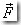is obtained as the vector product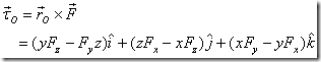where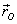is a vector from the point O to the point where the force is being applied. Actuallycould be a vector from O to any point along the line of action of the force as we will see below. The magnitude of the torque is given as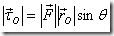Thus the magnitude of torque is equal to the product of the magnitude of the force and the perpendicular distance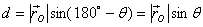from O to the line of action of the force as shown in figure 7 in the plane containing point O and the force vector. Since this distance is fixed, the torque due to a force can be calculated by taking vectorto be any vector from O to the line of action of the force. The unit of a torque is Newton-meter or simply Nm.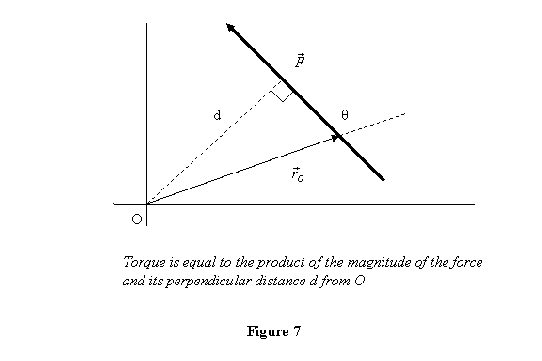### One Response to “Engineering Mechanics | Torque due to a force”

1.great job!!
:))